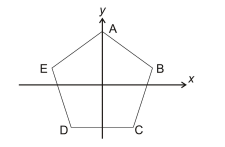# IMAT 2012 Q78 [Transformations]

ABCDE is a regular pentagon. The transformation R is a rotation about the origin and maps A to B, B to C, C to D, etc. The transformation S is a reflection in the y-­axis.Which of the following sequences of transformations (performed in the order that they are listed) would NOT leave vertex D in the same position?

A. R R S R S R
B. S R
C. S R R S R S
D. R S R S
E. R S R R

The answer is A, sequence R R S R S R.

• By undergoing R twice, we are at back at A.

• By undergoing S, this leaves us still at A.

• By undergoing R once again, we are now at B.

• By undergoing S, we have completely left the pentagon and another R nor S can put us back on the pentagon respectively.

Therefore, A will not leave D at it’s start position.